## Halley's Irrational Formula

A Root-finding Algorithm which makes use of a third-order Taylor Series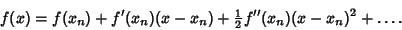(1)

A Root ofsatisfies, so(2)

Using the Quadratic Equation then gives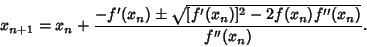(3)

Picking the plus sign gives the iteration function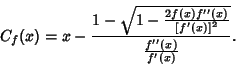(4)

This equation can be used as a starting point for deriving Halley's Method.

If the alternate form of the Quadratic Equation is used instead in solving (2), the iteration function becomes instead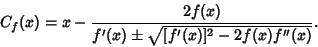(5)

This form can also be derived by setting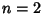in Laguerre's Method. Numerically, the Sign in the Denominator is chosen to maximize its Absolute Value. Note that in the above equation, if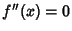, then Newton's Method is recovered. This form of Halley's irrational formula has cubic convergence, and is usually found to be substantially more stable than Newton's Method. However, it does run into difficulty when bothand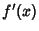orand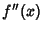are simultaneously near zero.

Qiu, H. A Robust Examination of the Newton-Raphson Method with Strong Global Convergence Properties.'' Master's Thesis. University of Central Florida, 1993.
Scavo, T. R. and Thoo, J. B. On the Geometry of Halley's Method.'' Amer. Math. Monthly 102, 417-426, 1995.